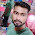### Program 168:To Interchange of Diagonals in Matrix

Program 168:

```#include<stdio.h>
main()
{
int i,j,rows,col,temp;
printf("Enter number of rows\n");
scanf("%d %d",&rows,&col);

int a[rows][col];
if(col==rows)
{
//Taking input for matrix
printf("Enter Matrix 1\n");
for(i=0;i<rows;i++)
{
for(j=0;j<col;j++)
{
scanf("%d",&a[i][j]);
}
}
printf("The given Matrix is\n");
for(i=0;i<rows;i++)
{
for(j=0;j<col;j++)
{
printf("%d\t",a[i][j]);
}
printf("\n");
}

for(i=0;i<rows;i++)
{
temp=a[i][i];
a[i][i]=a[i][rows-i-1];
a[i][rows-i-1]=temp;

}

printf("The Matrix After Interchanging Diagonals is\n");
for(i=0;i<rows;i++)
{
for(j=0;j<col;j++)
{
printf("%d\t",a[i][j]);
}
printf("\n");
}
}
else
{
printf("Not Possible\n");
}

}```
Explanation:

//Coming Soon...

Output:

#### 1 comment:

1.factorial hundred In the last few days, the “factorial of 100” is one of the top subjects and a lot of maths geeks compute it using voice assistants such as Alexa, Shiri, etc.

factorial hundred In the last few days, the “factorial of 100” is one of the top subjects and a lot of maths geeks compute it using voice assistants such as Alexa, Shiri, etc.

factorial hundred In the last few days, the “factorial of 100” is one of the top subjects and a lot of maths geeks compute it using voice assistants such as Alexa, Shiri, etc.

 Donate Buy me a coffee \$2.00 USD Buy me a burger \$5.00 USD Buy me a pizza \$10.00 USDCompiler Used by me C-Free 5.0(Recommended) Other Compilers Code Blocks(Recommended) Online Compilers Ideone(Remember to give input before executing online where ever necessary)